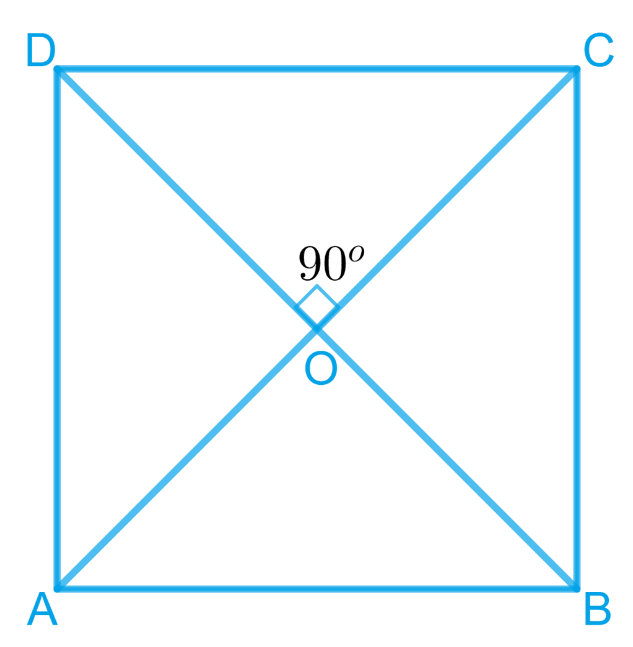In the verge of coronavirus pandemic, we are providing FREE access to our entire Online Curriculum to ensure Learning Doesn't STOP!

# Ex.8.1 Q5 Quadrilaterals Solution - NCERT Maths Class 9

Go back to  'Ex.8.1'

## Question

Show that if the diagonals of a quadrilateral are equal and bisect each other at right angles, then it is a square.

Video Solution
Ex 8.1 | Question 5

## Text Solution

What is known?

The diagonals of a quadrilateral are equal and bisect each other at right angle.

What is unknown?

How we can show that it is a square.

Reasoning:

We have to show that given quadrilateral is a parallelogram in which all sides are equal and one of its interior angles is .Steps:

Let us consider a quadrilateral $$ABCD$$ in which the diagonals $$AC$$ and $$BD$$ intersect each other at $$O$$. It is given that the diagonals of $$ABCD$$ are equal and bisect each other at right angles.

Therefore,

$${\rm{AC }} = {\rm{ BD}},{\rm{ OA }} = {\rm{ OC}},{\rm{ OB }} = {\rm{ OD}},$$ and

$$\angle \,{\rm{AOB }} \!=\!\angle {\rm{BOC }} \!=\! \angle {\rm{COD }} \!=\! \angle {\rm{AOD }} \!=\! {\rm{ 9}}{0^0}$$

To prove $$ABCD$$ is a square

We have to prove that $$ABCD$$ is a parallelogram,in which

$$AB = BC = CD = AD$$, and one of its interior angles is $$90^{0}$$ .

In $${ \Delta{AOD} }{ {\: \rm and } \:\Delta {COD}}$$

\begin{align}{{AO}}&={CO}\\ \text{(Diagonals }& \text { bisect each other)} \\\\ \angle {AOD}&=\angle {COD}\\text{ Given that} &\text{ each is } 90^{\circ}) \\\\ {{OD}}&={{OD}\quad( \text{ Common })}\\ \therefore \Delta {AOD} &\cong \Delta {COD}\\ \text{(SAS}& \text { congruence rule)} \\\\ \therefore {AD}&={{DC}}\quad\dots(3)\end{align} $\rm {And,}\angle \mathrm{OAB}=\angle \mathrm{OCD}(\mathrm{By} \,\text {CPCT})$ However, these are alternate interior angles for line \(AB and $$CD$$ and alternate interior angles are equal to each other only when the two lines are parallel.

$$AB \;|| \;CD$$... (2)

From Equations (1) and (2), we obtain $$ABCD$$ is a parallelogram.

In $$\Delta AOD$$ and $$\Delta {COD}$$

\begin{align} {{AO}}&={CO}\\ \text{(Diagonals }& \text { bisect each other)} \\ \\\ {\angle {AOD}}&=\angle {COD}\\text{ Given that} &\text{ each is } 90^{\circ})\\\\ {{OD}}&={{OD}\,\,( \text{ Common })}\\ \therefore \Delta {AOD} &\cong \Delta {COD}\\ \text{(SAS}& \text { congruence rule)} \\\\ \therefore {AD}&={{DC}}\qquad\dots(3)\end{align} However, \(AD = BC and $$AB = CD$$

(Opposite sides of parallelogram $$ABCD$$)

$$AB = BC = CD = DA$$

Therefore, all the sides of quadrilateral $$ABCD$$ are equal to each other.

In $$\Delta {ADC}$$ and $$\Delta {BCD} ,$$

\begin{align}\\ {{AD}}&={{BC}\; ( \text{ Already proved })} \\ {{AC}}&={{BD} \;\text{ (Given) }} \\ {{DC}}&={{CD}\; ( \text{ Common })}\\{\therefore \Delta {ADC} }&\cong \Delta {BCD}\\ \text{(SAS}& \text { congruence rule)} \\\\ {\therefore\angle {ADC}}&={\angle {BCD}\;(\text{By} \,\text{CPCT})}\end{align}

However,

\begin{align} \angle {ADC}\!+\angle {BCD}&=180^{\circ}\\ \text{ (Co-interior }& \text {angles) } \\\\ {\qquad \angle {ADC}+\angle {ADC}}&={180^{\circ}}\\{2 \angle {ADC}}&={180^{\circ}} \\ {\therefore \angle {ADC}}&={90^{\circ}}\end{align}

One of the interior angles of quadrilateral $$ABCD$$ is a right angle.

Thus, we have obtained that $$ABCD$$ is a parallelogram, $$AB = BC = CD = AD$$ and one of its interior angles is $$90^{\circ}$$ .

Therefore, $$ABCD$$ is a square.

Video Solution